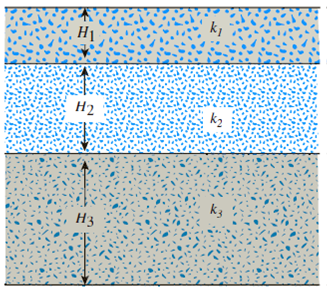Chapter 7, Problem 7.21PPrinciples of Geotechnical Enginee...

9th Edition
Braja M. Das + 1 other
ISBN: 9781305970939

Solutions

Chapter
SectionPrinciples of Geotechnical Enginee...

9th Edition
Braja M. Das + 1 other
ISBN: 9781305970939
Textbook Problem

A layered soil is shown in Figure 7.33. Given:• H1 = 1.5 m k1 = 9 × 104 cm/sec• H2 = 2.5 m k2 = 7.8 × 10−3 cm/sec• H3 = 3.5 m k3 = 4.5 × 10−5 cm/secEstimate the ratio of equivalent permeability, kH(eq)/kV(eq).Figure 7.33

To determine

Find the ratio of equivalent permeability kH(eq)kV(eq).

Explanation

Given information:

The depth of first layer H1 is 1.5 cm.

The hydraulic conductivity for first layer (k1) is 9×104cm/sec.

The depth of second layer H2 is 2.5 cm.

The hydraulic conductivity (k2) second layer is 7.8×103cm/sec.

The depth of third layer H3 is 3.5 cm.

The hydraulic conductivity (k3) third layer is 4.5×105cm/sec.

Calculation:

Determine the hydraulic conductivity in the horizontal direction using the relation.

kH(eq)=1H(k1H1+k2H2+k3H3)

Here, H is the total depth of the soil layer.

Substitute 7.5 cm for H, 9×104cm/sec for k1, 1.5 cm for H1, 7.8×103cm/sec for k2, 2.5 cm for H2, 3.5 cm for H3, and 4.5×105cm/sec for k1.

kH(eq)=17.5(9×104×1.5+7.8×103×2.5+4.5×105×3.5)=17.5(0.00135+0.0195+0.0001575)=2.801×103cm/sec

Determine the hydraulic conductivity in the vertical direction using the relation.

kV(eq)=HH1k1+H2k2+H3k3

Substitute 7

Still sussing out bartleby?

Check out a sample textbook solution.

See a sample solution

The Solution to Your Study Problems

Bartleby provides explanations to thousands of textbook problems written by our experts, many with advanced degrees!

Get Started

Find more solutions based on key concepts

Principles of Information Systems (MindTap Course List)

What is total cost of ownership (TCO)? What costs often are underestimated?

Systems Analysis and Design (Shelly Cashman Series) (MindTap Course List)

What is hot work?

Welding: Principles and Applications (MindTap Course List)

Compare the price and quality of laser printers to ink-jet printers.

Enhanced Discovering Computers 2017 (Shelly Cashman Series) (MindTap Course List)